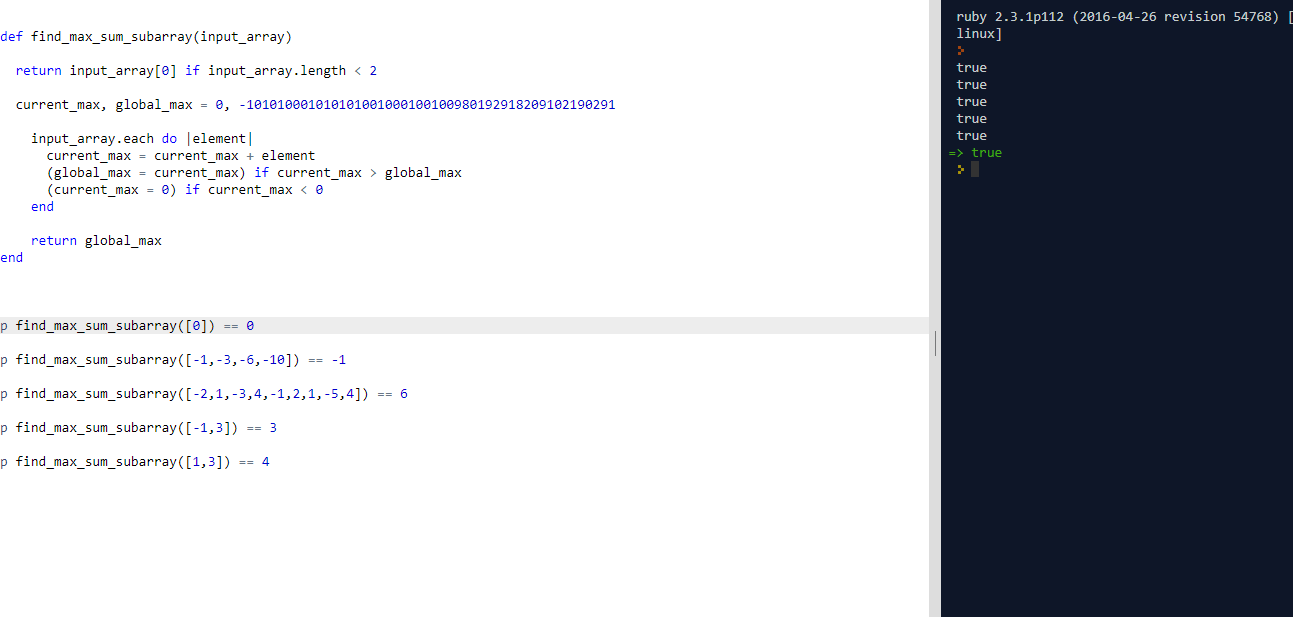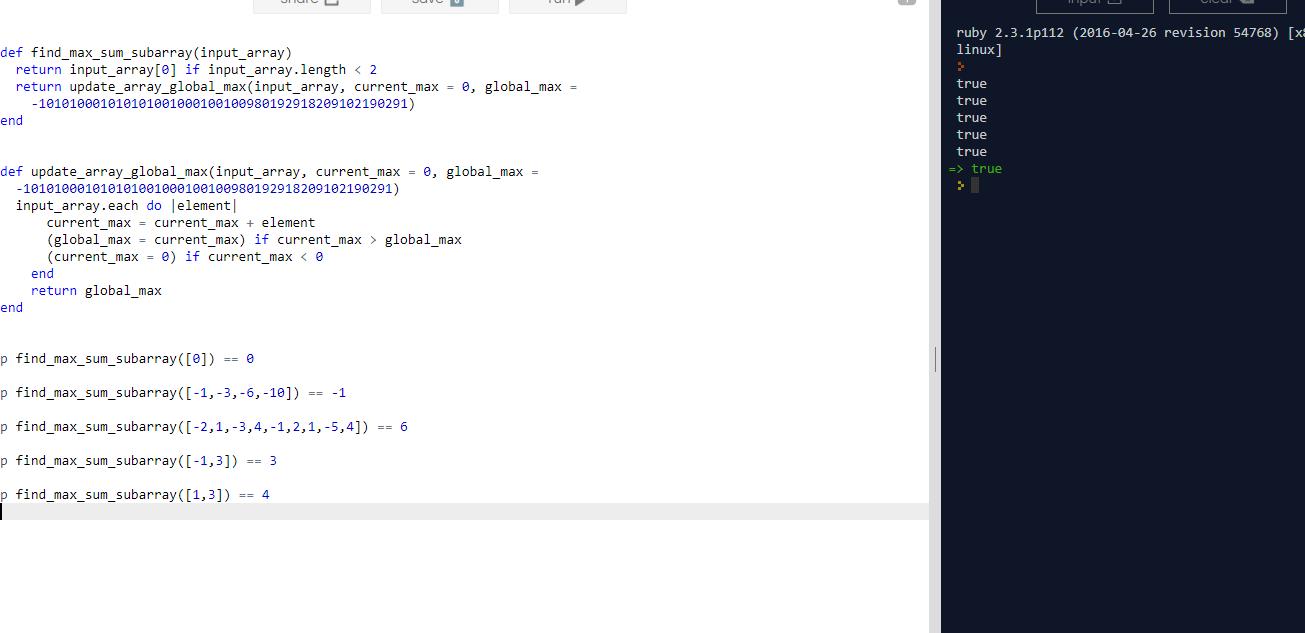# (*) CLEAN CODE: FIND THE CONTIGUOUS SUBARRAY WITHIN AN ARRAY (CONTAINING AT LEAST ONE NUMBER) WHICH HAS THE LARGEST SUM.

By: Saurav

2017-11-06 09:11:00 UTC

Question: Find the contiguous subarray within an array (containing at least one number) which has the largest sum.

For example:

Given the array [-2,1,-3,4,-1,2,1,-5,4],

the contiguous subarray [4,-1,2,1] has the largest sum = 6.

For this problem, return the maximum sum.

InterviewBit

Solution:
A really beautiful approach to the problem is to iterate the array, take sum and not carry negative sum results for the next iteration. If we already know that we got negative result in evaluating the current sum till previous element, why bring it to the next iteration if its addition only forces new sum to go more leftwards on the number line ?

But its important to store that current sum in max sum till previous element for the case of next element being more negative.

Attempt 1:

```def find_max_sum_subarray(input_array)

return input_array if input_array.length < 2

current_max, global_max = 0, -101010001010101001000100100980192918209102190291

input_array.each do |element|
current_max = current_max + element
(global_max = current_max) if current_max > global_max
(current_max = 0) if current_max < 0
end

return global_max
end

p find_max_sum_subarray() == 0

p find_max_sum_subarray([-1,-3,-6,-10]) == -1

p find_max_sum_subarray([-2,1,-3,4,-1,2,1,-5,4]) == 6

p find_max_sum_subarray([-1,3]) == 3

p find_max_sum_subarray([1,3]) == 4
```As we can see here, all the tests pass.

Now its time for refactoring:

Refactoring:

```def find_max_sum_subarray(input_array)
return input_array if input_array.length < 2
return update_array_global_max(input_array)
end

private

def update_array_global_max(input_array, current_max = 0, global_max = -101010001010101001000100100980192918209102190291)
input_array.each do |element|
current_max = current_max + element
(global_max = current_max) if current_max > global_max
(current_max = 0) if current_max < 0
end
return global_max
end

p find_max_sum_subarray() == 0

p find_max_sum_subarray([-1,-3,-6,-10]) == -1

p find_max_sum_subarray([-2,1,-3,4,-1,2,1,-5,4]) == 6

p find_max_sum_subarray([-1,3]) == 3

p find_max_sum_subarray([1,3]) == 4
```

Breaking down the problem and following the clean code book recommendation of "a function should do just one thing", having two functions that do their single task causes the code to be way cleaner.

By Including private here, I meant to indicate that these method would be part of a class and only one would be allowed in the public interface for other objects to send and receive messages to.

As we can see, all tests pass and we so we can rest for a while :)Its great to share with others! One of my friends Srikiran Sistla suggested a cleaner way of doing this:

In this case, we carry the value from previous iteration for contiguous sum, and add it to the next value. If the value at that position is greater than that sum, we drop the last sum and set the sum to the new array element and start again. That way, if the new value at that position is positive and the sum is negative, we only take the new positive one. If its negative and lesser than the total contiguous sum so far, we take the lesser value.

Its kind of the same in a way that we are updating the current value in two steps resetting it as zero in the last code and in this one we are doing it in one step only by directly setting it to the array element value.

```def find_max_sum_subarray(input_array)
return input_array if input_array.length < 2
return update_array_global_max(input_array)
end

private

def update_array_global_max(nums)
global_max = local_max = nums
(1..nums.size - 1).each do |i|
local_max = [local_max + nums[i], nums[i]].max
global_max = [local_max, global_max].max
end
return global_max
end

p find_max_sum_subarray() == 0

p find_max_sum_subarray([-1,-3,-6,-10]) == -1

p find_max_sum_subarray([-2,1,-3,4,-1,2,1,-5,4]) == 6

p find_max_sum_subarray([-1,3]) == 3

p find_max_sum_subarray([1,3]) == 4```

Owned & Maintained by Saurav Prakash

If you like what you see, you can help me cover server costs or buy me a cup of coffee though donation :)